# Triangles (and Trigonometry)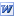Mathematics of a Total Solar Eclipse

A good 'similar triangles' activity to explain the shadow of a total solar eclipse. Harder examples of the main activity.Lesson planBulletpoints for lessonMore structured task for less experienced pupilsSupporting presentation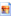SOHO image of SunEclipse mapSOHO websiteFour Questions on one page. PDF hereTriangle Flowchart

Flowchart for deciding which formula you need to use when doing questions on triangles.Pythagoras

A triangle with 3 squares around it, lengths 6cm, 8cm & 10cm. Print out, duplicate & have pupils cut out to fit the 2 smaller squares into the bigger one. Talk them through what they're doing to link it to Pythagoras' Theorem.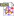Cabri File: Pythagoras

Dynamic version of Pythagoras. Move the points of the triangle around to see how the squares change too. This version was created by Mr Walsh. Instructions for how to make your own, similar, version are .How far is the horizon from any given height?

Pupils use Pythagoras to work out how far away the horizon is from any given altitude. This file sets up the mathematical model and then gives the answer too. Click for an easier, more numeric version. The answers and working to the numeric version.Trigonometry Basic Notes

Note on labelling the triangle and sin, cos, tan formula in speed/distance/time style triangles. PDF here.

We'll leave the rhymes for remembering sohcahtoa to you!Trigonometry

Lots and lots of differentiated trigonometry practice questions. Sorted into those requiring sin/cos vs those requiring tan, those requiring to find the hyp vs those requiring to find the opp/adj, those requiring to find the side vs those requiring to find the angle... Plus cosine and sine rule, 2D and 3D problems... Doesn't include answers but those that I've used are in an excel file.Trig Graphs

See how the graph & the fractions change as you manipulate the sides of the triangle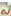Autograph File: Trig Graphs

Graph axes setup for producing sin / cos / tan graphs. Click for predrawn graphs, but its more fun doing it for yourself!Trig Graph Printout

Word doc of Sin / Cos / Tan graphs from -360 thru +720 to printout for pupils. Autograph files that mak up the document are here: Sin, Cos, Tan.Autograph File: Diagonal of a Cube

A 'dynamic' 3D visualization for helping pupils to see how to calculate the length of a diagonal of a cube given the length of its side.Seasons On Saturn

KS4 higher, application of standard (right-angle) trigonometry and transformations of curves.

This task involves students making and using measurements from Hubble Space Telescope images to determine Saturn’s orbital period and then transform the sin curve using the excel file to establish when it will next appear ‘edge-on’.  Lots of geometrical reasoning, problem solving and ICT.Saturn ImagesEdge-on LastSeasons on Saturn worksheetHubbles Views Diagram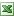Saturns Rings spreadsheet Hubbles Views Movie (1.2Mb)Saturn Trigonometry Animated Saturn All Essentials ZIPSin CurveLatest Image All Extras ZIPCosine Rule Triangle

Ppt file to help with labelling non-right-angled triangles for using the cosine and sine rule. Good for using with your interactive whiteboard - copy it into the notebook and rotate to get the sides labelled up correctly.